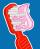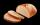Competition

The organizers of competion wanted to spent big amount of money to pay for the competitors. One third of this amount spent on prizes and the remaining € 5,000 to diplomas. How much was committed for rewards?

Result

x =  7500

Solution:

(1-1/3)*x = 5000

2x = 15000

x = 7500

Calculated by our simple equation calculator.

Leave us a comment of this math problem and its solution (i.e. if it is still somewhat unclear...):Be the first to comment!To solve this verbal math problem are needed these knowledge from mathematics:

Need help calculate sum, simplify or multiply fractions? Try our fraction calculator. Do you have a linear equation or system of equations and looking for its solution? Or do you have quadratic equation?

Next similar math problems:

1. HusseinHussein owns 450000. He spent at the bookstore 2 over 9 to buy some books and tales. He paid 3 over 5 of his money to buy his math book. Then he paid his money to buy stories diagram ; a. Calculate the remaining amount of money with Hussein? b. Hussein l
2. A manA man spends 5/9 of his money on rent, and 5/16 of the remainder on electricity. If the final balance remaining is 550 find how much was spent on rent
3. Chewing gumsFor 3 chewing gums, you will pay 20 CZK less than 7 chewing gums. How much is 1 chewing gum and how much does a 5pcs package cost?
4. Discount saleAfter the discount, the computer costs 9600, - CZK. How much did it cost when the price was reduced: a) by half b) by a third c) by one fifth and then by 160 CZK
5. Equation 20In given equation: 8/9-4/5=2/9+x, find x
6. ZdeněkZdeněk picked up 15 l of water from a 100-liter full-water barrel. Write a fraction of what part of Zdeněk's water he picked.
7. Mixed2improperWrite the mixed number as an improper fraction. 166 2/3
8. Simple equation 8Solve the following equation: 36=-(1+7x)-6(-7-x)
9. Fraction to decimalWrite the fraction 3/22 as a decimal.
10. In fractionsAn ant climbs 2/5 of the pole on the first hour and climbs 1/4 of the pole on the next hour. What part of the pole does the ant climb in two hours?
11. Simple equation 5Solve equation with fractions: X × 3/8 = 1/2
12. Forest nurseryIn the forest nursery after winter, they found that 1/10 stems died out of them. For them, they land 193 new spruces. How many spruces are in the forest nursery?
13. Lengths of the poolMiguel swam 6 lengths of the pool. Mat swam 3 times as far as Miguel. Lionel swam 1/3 as far as Miguel. How many lengths did mat swim?
14. Fraction and a decimalWrite as a fraction and a decimal. One and two plus three and five hundredths
15. Unknown numberDetermine the unknown number that is equal to a quarter of the fifth of number, which is by 152 more than unknown number.Several pieces of bread were in the store. Half of them plus a half of bread bought a cookbook. Of the remaining bread, half of it plus half of the bread was bought by Mr. Novák. The last bread was bought by Ms. Small. How many breads were in the store at.One-eighth of 9th class was interested in studying at a grammar school, at a business academy one sixth, at secondary vocational schools quarter, to SOU one third and the remaining three students were interested in the school of art direction. How many st